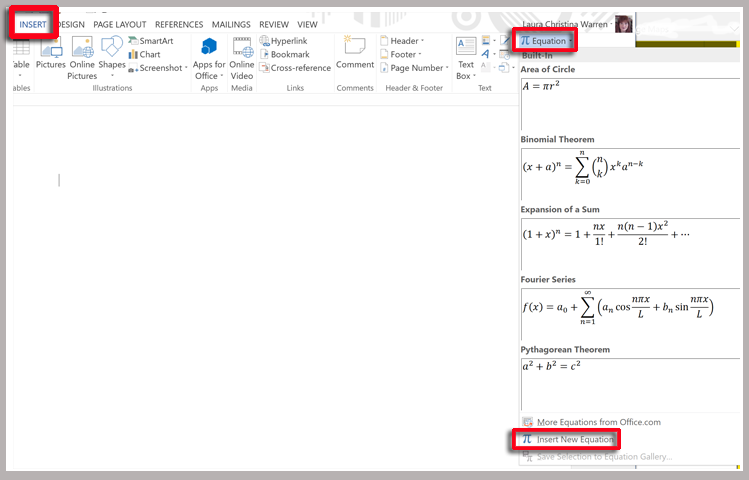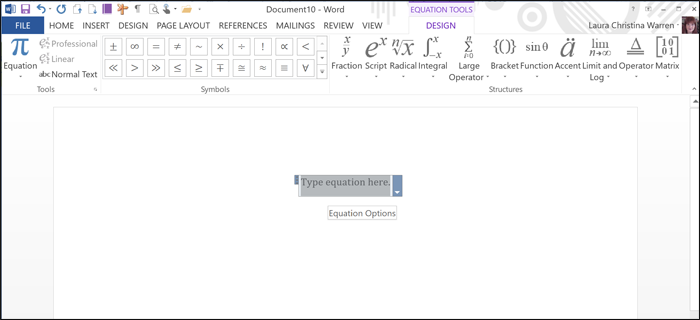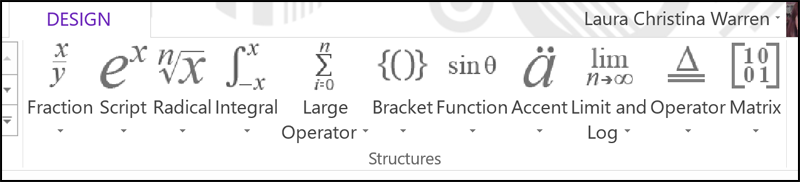Select Page

Share

If you need to insert an equation in Word, position your cursor where you want the equation to appear and go to the Insert tab.  If you click on the arrow to the right of Equation, a group of built-in equations will appear.If you don’t see what you want, click on Insert New Equation at the bottom.   Alternately, you could have pressed Alt+= and immediately the Equation Tools – Design tab appears with a blank equation field.If you chose to press Alt+= rather then Insert > Equation, the Equation drop-down list is at the extreme left, where you can choose from a list of equations.

If you build your own equation, you can use a variety of symbols in several categories ……as well as Structures to click on as you build your equation.Once your equation is completed you can click on the arrow to the right of the equation field to display a group of options.• If you select Save as New Equation, your equation will be included in the Equation drop-down list.
• If you select Professional, your equation will not be shown on one line, as opposed to Linear.
• If you select Linear, it will be represented on one line (supports Unicode and LaTeX math).
• If you select Change to Inline, the equation will be treated as a single character within your text.  Otherwise, your equation will be centered.
• If you select Justification, you can set the alignment of the equation.  The default is centered.